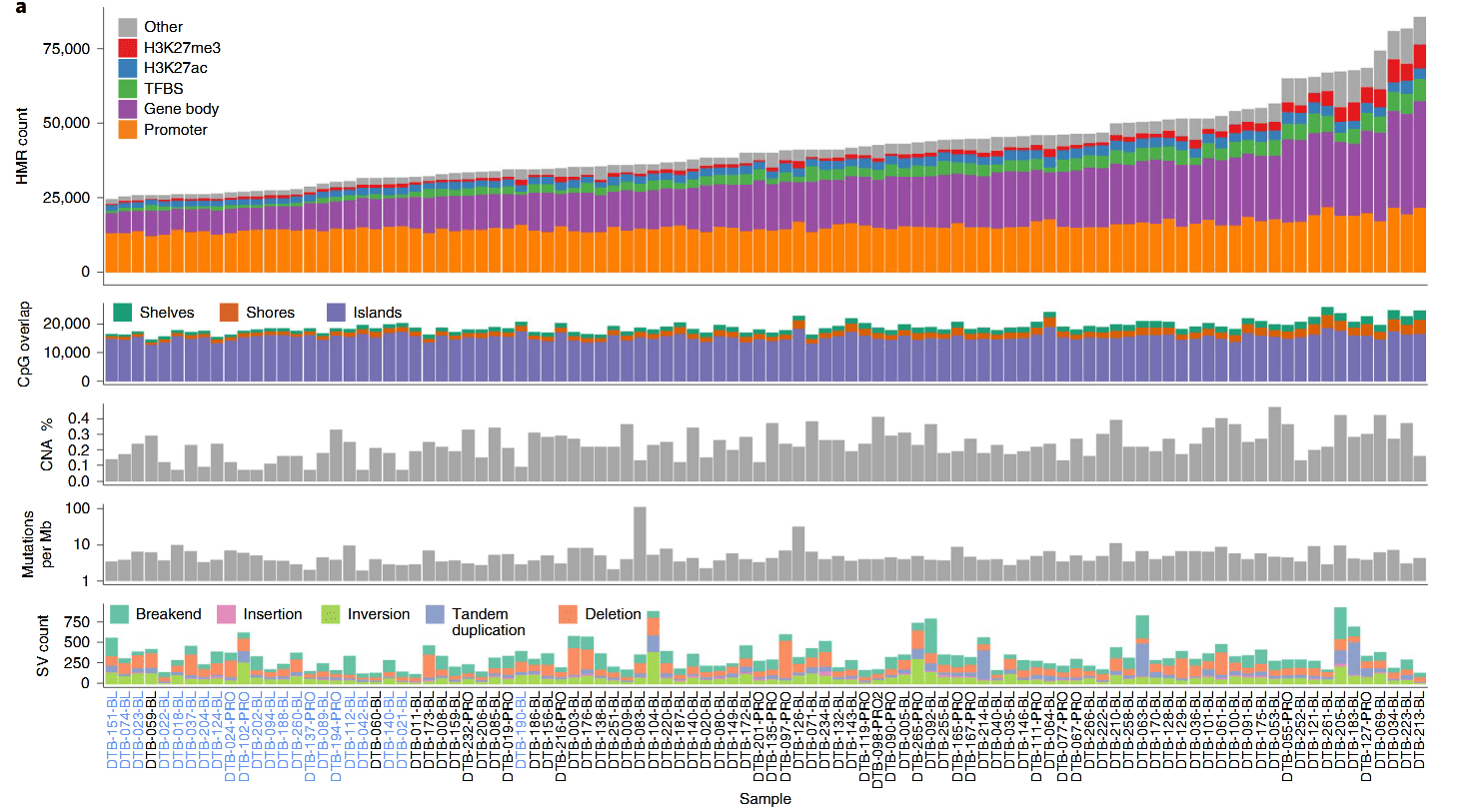# How to draw multiple images into one image in R language

#### Introduction

Sometimes we need to arrange multiple images on a picture. For the functions of the R language basic package, such as plot, barplot, hist, etc., it can be typeset through par, layout, but for ggplot images, we need Use another method

##### Several methods to achieve
###### par method

The method only applies to the functions of the basic package (plot, barplot, hist, etc.), not to ggplot.``````par(mfrow = c(1,2))
plot(mtcars[,1:2],pch =19)
plot(iris[,1:2],pch = 19)``````
###### layout method
``layout(matrix(c(2,0,1,3),2,2,byrow=TRUE), widths=c(3,1), heights=c(1,3), respect=TRUE)``

Set the layout, the layout matrix is

``````2  0
1  3``````

It means that the lower left is the first painting, the upper left is the second, the lower right is the third, and the upper right is not drawn. widths = c(3,1) means that the width ratio from left to right is 3 to 1, heights=c(1,3) means that the height is 1 to three from top to bottom, respect=True unit column width and unit row height The same physical measurement on the device

The example is as follows``````#Read in data

#First set the layout
layout(matrix(c(2,0,1,3),2,2,byrow=TRUE), widths=c(3,1), heights=c(1,3), TRUE)

#Draw a scatter plot
par(mar=c(5.1,4.1,0.1,0))
plot(air\$Respirable.Particles~air\$Nitrogen.Oxides,pch=19,
col="black",xlim=c(0,600),ylim=c(0,80),xlab="Nitrogen Oxides Concentrations",ylab="Respirable Particle Concentrations")

#Draw the histogram of X, above the scatter plot
par(mar=c(0,4.1,3,0))
hist(air\$Nitrogen.Oxides,breaks=seq(0,600,100),ann=FALSE,axes=FALSE,
col="black",border="white")

#Draw a histogram of Y, to the right of the scatter plot
yhist <- hist(air\$Respirable.Particles,breaks=seq(0,80,10),plot=FALSE)
par(mar=c(5.1,0,0.1,1))
barplot(yhist\$density,horiz=TRUE,space=0,axes=FALSE,col="black",border="white")``````
###### ggplot can use the function ggarange of the ggpubr package

Use install.packages("ggpubr") to install the ggpubr package.

``````ggarrange(...,
plotlist = NULL,
ncol = NULL,
nrow = NULL,
labels = NULL,
label.x = 0,
label.y = 1,
hjust = -0.5,
vjust = 1.5,
font.label = list(size = 14, color = "black", face = "bold", family = NULL),
align = c("none", "h", "v", "hv"),
widths = 1,
heights = 1,
legend = NULL,
common.legend = FALSE)``````

…：The figure object that needs to be drawn can be directly placed in multiple ggplot objects
plotlist，You can also put the ggplot object in the list and give this plotlist parameter
ncol and nrow are arranged in several rows and columns
labelsThe label of each figure, label.x label.y hjust vjust font.label is the position parameter of adjusting labels，
alignThe arrangement of each figure, is it vertical (v) or horizontal (h)，
widths and heights adjust the aspect ratio of each image
legend is the legend of each figure,
common.legend common legend

The example is as follows``````cols <- brewer.pal(n=5, name='Set1')  #Configure color palette
# Use plist to store ggplot objects, and then use ggarrange for typesetting and drawing
plist <- list()
plist[] <- ggplot(df1,aes(x=sample,y=count,fill=group))+theme_classic()+geom_bar(stat='identity')+
theme(legend.position = "none",
axis.title.x=element_blank(),
axis.text.x=element_blank(),
axis.ticks.x=element_blank()) +
ylab('HMR#')+
scale_fill_manual(values=c('darkgray',cols))
plist[] <- ggplot(df3,aes(x=sample,y=count,fill=group))+theme_classic()+geom_bar(stat='identity')+
theme(axis.title.x=element_blank(),axis.text.x=element_blank(),axis.ticks.x=element_blank(),legend.position = "none")+
scale_fill_brewer(palette='Dark2')+ylab('CpG#')
plist[] <- ggplot(df5,aes(x=sample,y=percent.copy.altered,fill=namecn))+theme_classic()+geom_bar(stat='identity')+
theme(axis.title.x=element_blank(),axis.text.x=element_blank(),axis.ticks.x=element_blank(),legend.position = "none")+
scale_fill_manual(values='darkgrey')+ylab('% CN Altered')
plist[] <- ggplot(df5,aes(x=sample,y=mutation_count,fill=namemut))+theme_classic()+geom_bar(stat='identity')+
theme(axis.title.x=element_blank(),axis.text.x=element_blank(),axis.ticks.x=element_blank(),legend.position = "none")+
scale_fill_manual(values='darkgrey')+ylab('Mut/Mb')+scale_y_log10()

plist[] <- ggplot(df6,aes(x=sample,y=count,fill=group))+theme_classic()+geom_bar(stat='identity')+
theme(axis.title.x=element_blank(),
axis.text.x=element_text(angle=90,hjust=1,vjust=0.5,colour=df6\$color),
axis.ticks.x=element_blank(),
legend.position = "none")+
scale_fill_brewer(palette='Set2')+ylab('# SV')

ggarrange(plotlist=plist,
ncol=1,
nrow=length(plist),
heights=c(3,1,1,1,2) ,
align="v")
ggsave(fn_fig1a, height=8, width=14)``````
###### ggplot can also use grid typesetting

The grid package is the underlying drawing system that comes with the R language, which can finely lay out graphs, lines, points, etc. We wrap it as a function here, and its usage is very similar to ggarrange.
Example：

``````# Multiple plot function
#
# ggplot objects can be passed in ..., or to plotlist (as a list of ggplot
# objects)
# - cols:   Number of columns in layout
# - layout: A matrix specifying the layout. If present, 'cols' is ignored.
#
# If the layout is something like matrix(c(1,2,3,3), nrow=2, byrow=TRUE),
# then plot 1 will go in the upper left, 2 will go in the upper right, and
# 3 will go all the way across the bottom.
# e=0.15, # extra height needed for last plot (vertical layout),
# or extra width for first plot (horizontal layout)
multiplot <- function(..., plotlist=NULL, file, cols=1,
layout=NULL, horizontal=FALSE, e=0.15) {
require(grid)

# Make a list from the ... arguments and plotlist
plots = c(list(...), plotlist)

numPlots = length(plots)
#message(paste0('>>>>>>>INFO: num plots 2 = ', numPlots), '\n')

# If layout is NULL, then use 'cols' to determine layout
if (is.null(layout)) {
# Make the panel
# ncol: Number of columns of plots
# nrow: Number of rows needed, calculated from # of cols
layout = matrix(seq(1, cols * ceiling(numPlots/cols)),
ncol = cols, nrow = ceiling(numPlots/cols))
}

if (numPlots==1) {
print(plots[])

} else {

## set up heights/widths of plots

# extra height needed for last plot (vertical layout),
# or extra width for first plot (horizontal layout)
hei = rep(1, numPlots)
# bottom plot is taller
hei[numPlots] = hei[numPlots]*(1+e)
wid = rep(1, numPlots)
# first left plot is wider
wid = wid*(1+e)
# Set up the page
grid.newpage()
if(horizontal){
pushViewport(viewport(layout = grid.layout(nrow(layout),
ncol(layout), widths=wid)))
}else{
pushViewport(viewport(layout = grid.layout(nrow(layout),
ncol(layout), heights=hei)))

}

# Make each plot, in the correct location
for (i in 1:numPlots) {
# Get i,j matrix positions of the regions containing this subplot
matchidx = as.data.frame(which(layout == i, arr.ind = TRUE))
print(plots[[i]], vp = viewport(layout.pos.row = matchidx\$row,
layout.pos.col = matchidx\$col))
}
}
}

library(ggplot2)
p1 <- ggplot(iris, aes(x = Sepal.Length)) + geom_histogram() + theme_bw()
p2 <- ggplot(iris, aes(x = Sepal.Length, y = Petal.Width)) + geom_point() + theme_bw()

# Use ggplot object to draw directly
multiplot(p1,p2)

# 将ggplot对象放入列表中，再用列表画图, 并设置两列的排列方式
plot_lst <- list()
plot_lst[] <- p1
plot_lst[] <- p2
multiplot(plotlist = plot_lst, cols = 2)``````

#### Reference

Hrishi V.Mittal, R Graphs Cookbook
Zhao S G , Chen W S , Li H , et al. The DNA methylation landscape of advanced prostate cancer[J]. Nature Genetics, 2020.
https://github.com/DavidQuigley/WCDT_WGBS/blob/master/scripts/2019_05_15_WGBS_figure_1A.R
Dang H X , White B S , Foltz S M , et al. ClonEvol: clonal ordering and visualization in cancer sequencing[J]. Annals of Oncology, 2017.
ywliao blog： https://www.cnblogs.com/ywliao/p/12419025.html